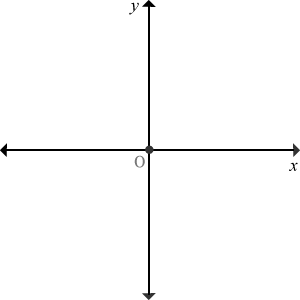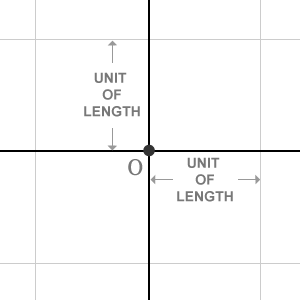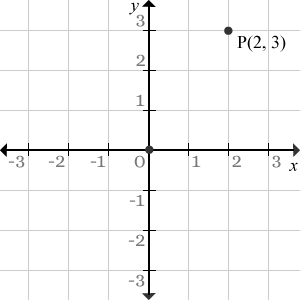# Bi Dimensional Cartesian coordinate system

A geometric coordinate system of displaying position of a point in a plane from two different perpendicular bisecting reference lines, is defined two dimensional Cartesian coordinate system (2D Cartesian coordinate system).

Two dimensional Cartesian coordinate system is also known as Bi-dimensional Cartesian coordinate system. Similarly, it also simply called as two dimensional space or bi-dimensional space. Two dimensional space is most commonly used coordinate system in geometry. It is mainly used to identify the exact location of a point in the plane. Before going to do it, understand the construction of the bi-dimensional space in geometry.

## Construction

Consider a plane and draw a horizontal straight line exactly middle to the plane. Also draw another straight line exactly middle to the same plane but it is perpendicular to the horizontal line.Thus, they both are interested at a point and it divided entire plane into four equal parts. The intersecting point is called origin. It is usually denoted by $O$. The horizontal line is called as $x$-axis and the vertical line is called as $y$-axis in the plane.

In this 2D Cartesian coordinate system, the location of the every point on the plane is expressed in terms of the distance of the point from the $x$-axis and also distance of the point from the $y$-axis. The distance of the point from $x$-axis is known as $x$-coordinate and the distance of the same point from $y$-axis is also known as $y$-coordinate. They both are expressed by a comma separation inside the parenthesis to represent the exact location of the point in mathematics.

For example, the origin $O$ is on the $x$-axis and also on the $y$-axis, which means there is no distance between point $O$ and the $x$-axis, and there is also no distance from origin to $y$-axis. So, the distances of the point from $x$-axis and from $y$-axis are $0$ and $0$ respectively. Therefore, the location of the origin in 2d Cartesian coordinate system is $O\left(0,0\right)$.In order to express every point on the plane in geometric coordinates form in Cartesian coordinate system, divide the entire plane into numerous equal parts horizontally and also divide the same plane into same equal parts vertically. Here, the plane is divided into few parts as displayed in the above first graphical presentation of two-dimensional space for your better understanding. It is zoomed in second image. Each horizontal division on $x$-axis and every vertical division on $y$-axis is known as a unit of length. The concept of unit of length is really useful to measure the distances of every point from both $x$-axis and $y$-axis and they can be written in mathematics in ordered pair form.

### How to useIn Bi-dimensional space, real numbers are used to represent length of the each division on both $x$-axis and y-axis. The divisions of the right side part of the $x$-axis and left side part of the $x$-axis are represented by the positive and negative real numbers respectively. Similarly, the division of the upper part of the $y$-axis and lower part of $y$-axis are represented by the positive and negative real numbers. In this example, integers are used to represent every division on every axis because integers are also real numbers. Therefore, $x$-axis and $y$-axis are used as reference lines to identify the point on the plane exactly.

Example

Consider a point on this plane and assume it is located at $2$ units distance from the $y$-axis and $3$ units distance from the $x$-axis. Assume, the name of the point is $P$. The distance of the point from $y$-axis is called $x$-coordinate and the distance of the point from $x$-axis is known as $y$-coordinate. So, the location of the point $P$ is $P\left(2,3\right)$. In this example, the unit of length is $1$. Due to this, the value of the every division is increased by $1$ on both axes.

Latest Math Topics
Latest Math Problems
Email subscription
Math Doubts is a best place to learn mathematics and from basics to advanced scientific level for students, teachers and researchers. Know more
Follow us on Social Media
###### Math Problems

Learn how to solve easy to difficult mathematics problems of all topics in various methods with step by step process and also maths questions for practising.

Learn more